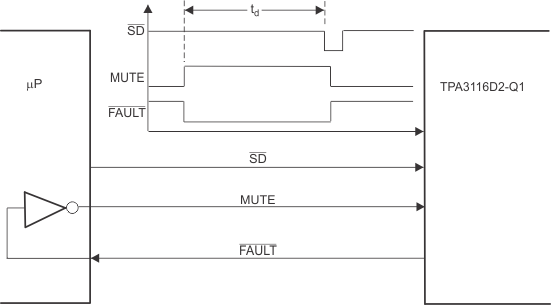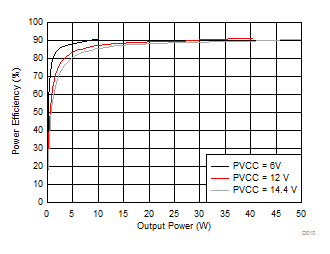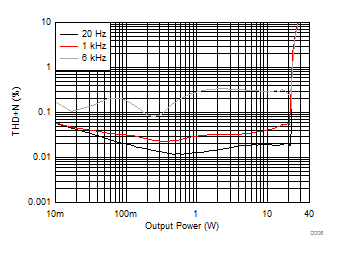SLOS862B July   2015  – October 2016

PRODUCTION DATA.

1. Features
2. Applications
3. Description
4. Revision History
5. Pin Configuration and Functions
6. Specifications
7. Detailed Description
1. 7.1 Overview
2. 7.2 Functional Block Diagram
3. 7.3 Feature Description
4. 7.4 Device Functional Mode
8. Application and Implementation
1. 8.1 Application Information
2. 8.2 Typical Application
9. Power Supply Recommendations
10. 10Layout
11. 11Device and Documentation Support
12. 12Mechanical, Packaging, and Orderable Information

• DAP|32
• DAP|32

## 6 Specifications

### 6.1 Absolute Maximum Ratings

over operating ambient temperature range (unless otherwise noted)(1)
MIN MAX UNIT
Supply voltage, VCC PVCC, AVCC –0.3 30 V
Input voltage, VI INPL, INNL, INPR, INNR –0.3 6.3 V
PLIMIT, GAIN/SLV, SYNC –0.3 GVDD + 0.3 V
AM0, AM1, AM2, MUTE, SD, MODSEL –0.3 PVCC + 0.3 V
Slew rate, maximum(2) AM0, AM1, AM2, MUTE, SD, MODSEL 10 V/ms
Operating ambient temperature, TA –40 125 °C
Operating junction temperature range, TJ –40 150 °C
Storage temperature range, Tstg –40 125 °C
(1) Stresses beyond those listed under Absolute Maximum Ratings may cause permanent damage to the device. These are stress ratings only, and functional operation of the device at these or any other conditions beyond those indicated under Recommended Operating Conditions is not implied. Exposure to absolute-maximum-rated conditions for extended periods may affect device reliability.
(2) 100-kΩ series resistor is needed if maximum slew rate is exceeded.

### 6.2 ESD Ratings

VALUE UNIT
V(ESD) Electrostatic discharge Human-body model (HBM), per AEC Q100-002(1) ±2000 V
Charged-device model (CDM), per AEC Q100-011 All pins ±450
Corner pins (1, 16, 17, and 32) ±450
(1) AEC Q100-002 indicates that HBM stressing shall be in accordance with the ANSI/ESDA/JEDEC JS-001 specification.

### 6.3 Recommended Operating Conditions

over operating ambient temperature range (unless otherwise noted)
MIN NOM MAX UNIT
VCC Supply voltage PVCC, AVCC 4.5 26 V
VIH High-level input voltage AM0, AM1, AM2, MUTE, SD, SYNC, MODSEL 2 V
VIL Low-level input voltage AM0, AM1, AM2, MUTE, SD, SYNC, MODSEL 0.8 V
VOL Low-level output voltage FAULT, RPULLUP = 100 kΩ, V(PVCC) = 26 V 0.8 V
IIH High-level input current AM0, AM1, AM2, MUTE, SD, MODSEL (VI = 2 V, VCC = 18 V) 50 µA
RL Minimum load impedance Output filter: L = 10 µH, C = 680 nF, BTL 3.2 4 Ω
Output filter: L = 10 µH, C = 1 µF, PBTL 1.6
Lo Output-filter inductance Minimum output filter inductance under short-circuit condition 1 µH

### 6.4 Thermal Information

THERMAL METRIC(1) TPA3116D2-Q1 TPA3118D2-Q1 UNIT
32 PINS 32 PINS
RθJA Junction-to-ambient thermal resistance 44.7(2) 32.4 °C/W
RθJC(top) Junction-to-case (top) thermal resistance 1.2 17.2 °C/W
RθJB Junction-to-board thermal resistance 21.5 17.3 °C/W
ψJT Junction-to-top characterization parameter 1.2 0.4 °C/W
ψJB Junction-to-board characterization parameter 21 17.2 °C/W
RθJC(bot) Junction-to-case (bottom) thermal resistance N/A 1 °C/W
(2) Modeled with a 15-mm × 15-mm × 2-mm copper heat slug heat sink. A better heat sink or airflow would yield a much better RθJA. Perfect heat sink results could be as low as RθJC(top) = 1.2 ºC/W.

### 6.5 DC Electrical Characteristics

TA = 25°C, AVCC = PVCC = 12 V to 24 V, RL = 4 Ω (unless otherwise noted)
PARAMETER TEST CONDITIONS MIN TYP MAX UNIT
| VOS | Class-D output offset voltage (measured differentially) VI = 0 V, gain = 36 dB 1.5 15 mV
ICC Quiescent supply current SD = 2 V, no load or filter, V(PVCC) = 12 V 20 35 mA
SD = 2 V, no load or filter, V(PVCC) = 24 V 32 50
ICC(SD) Quiescent supply current in shutdown mode SD = 0.8 V, no load or filter, V(PVCC) = 12 V <50 µA
SD = 0.8 V, no load or filter, V(PVCC) = 24 V 50 400
rDS(on) Drain-source on-state resistance, measured pin-to-pin V(PVCC) = 21 V, IO = 500 mA, TJ = 25°C 120
G Gain (BTL) R1 = open, R2 = 20 kΩ 19 20 21 dB
R1 = 100 kΩ, R2 = 20 kΩ 25 26 27
R1 = 100 kΩ, R2 = 39 kΩ 31 32 33 dB
R1 = 75 kΩ, R2 = 47 kΩ 35 36 37
G Gain (SLV) R1 = 51 kΩ, R2 = 51 kΩ 19 20 21 dB
R1 = 47 kΩ, R2 = 75 kΩ 25 26 27
R1 = 39 kΩ, R2 = 100 kΩ 31 32 33 dB
R1 = 16 kΩ, R2 = 100 kΩ 35 36 37
ton Turn-on time V(SD) = 2 V 10 ms
toff Turn-off time V(SD) = 0.8 V 2 µs
GVDD Gate drive supply I(GVDD) < 200 µA 6.4 6.9 7.4 V
VO Output voltage maximum under PLIMIT control V(PLIMIT) = 2 V; VI = 1 Vrms 6.75 7.9 8.75 V

### 6.6 AC Electrical Characteristics

TA = 25°C, AVCC = PVCC = 12 V to 24 V, RL = 4 Ω (unless otherwise noted)
PARAMETER TEST CONDITIONS MIN TYP MAX UNIT
KSVR Power supply ripple rejection 200 mVPP ripple at 1 kHz, gain = 20 dB, inputs ac-coupled to GND –70 dB
PO Continuous output power THD+N = 10%, f = 1 kHz, V(PVCC) = 14.4 V 25 W
THD+N = 10%, f = 1 kHz, V(PVCC) = 21 V 50
THD+N Total harmonic distortion + noise VCC = 21 V, f = 1 kHz, PO = 25 W (half-power) 0.1%
Vn Output integrated noise 20 Hz to 22 kHz, A-weighted filter, gain = 20 dB 65 µV
–80 dBV
Crosstalk VO = 1 Vrms, gain = 20 dB, f = 1 kHz –100 dB
SNR Signal-to-noise ratio Maximum output at THD+N < 1%, f = 1 kHz, gain = 20 dB, A-weighted 102 dB
fOSC Oscillator frequency AM[2:0] = 000 376 400 424 kHz
AM[2:0] = 001 470 500 530
AM[2:0] = 010 564 600 636
AM[2:0] = 011 940 1000 1060
AM[2:0] = 100 1128 1200 1278
AM[2:0] = 101 Reserved
AM[2:0] = 110
AM[2:0] = 111
Thermal trip point 150 °C
Thermal hysteresis 15 °C
Overcurrent trip point 7.5 A

### 6.7 Timing Requirements

MIN NOM MAX UNIT
td Delay from MUTE rising to SD falling 1.4 sFigure 1. Timing Requirement for SD

### 6.8 Typical Characteristics

fs = 400 kHz, BD mode (unless otherwise noted)Gain = 26 dB PVCC = 6 V TA = 25°C RL = 4 Ω 10-µH + 0.68-µF filter
Figure 2. Total Harmonic Distortion + Noise (BTL) vs FrequencyGain = 26 dB PVCC = 6 V TA = 25°C RL = 4 Ω 10-µH + 0.68-µF filter
Figure 4. Total Harmonic Distortion + Noise (BTL) vs Output PowerGain = 26 dB PVCC = 14.4 V TA = 25°C RL = 4 Ω 10-µH + 0.68-µF filter
Figure 6. Output Power (BTL) vs PLIMIT VoltageGain = 26 dB TA = 25°C RL = 4 Ω 10-µH + 0.68-µF filter
Figure 8. Power Efficiency (BTL) vs Output PowerGain = 26 dB PVCC = 14.4 V TA = 25°C RL = 4 Ω 22-µH + 1-µF filter
Figure 10. Total Harmonic Distortion + Noise (BTL) vs FrequencyGain = 26 dB PVCC = 14.4 V TA = 25°C RL = 4 Ω 10-µH + 0.68-µF filter
Figure 3. Total Harmonic Distortion + Noise (BTL) vs FrequencyGain = 26 dB PVCC = 14.4 V TA = 25°C RL = 4 Ω 10-µH + 0.68-µF filter
Figure 5. Total Harmonic Distortion + Noise (BTL) vs Output PowerGain = 26 dB TA = 25°C RL = 4 Ω 10-µH + 0.68-µF filter
Figure 7. Maximum Output Power (BTL) vs Supply VoltageGain = 26 dB PVCC = 12 V TA = 25°C RL = 4 Ω 10-µH + 0.68-µF filter
Figure 9. Crosstalk vs FrequencyPVCC = 14.4 V TA = 25°C RL = 4 Ω 22-µH + 3.3-µF filter
Figure 11. Gain and Phase (BTL) vs Frequency